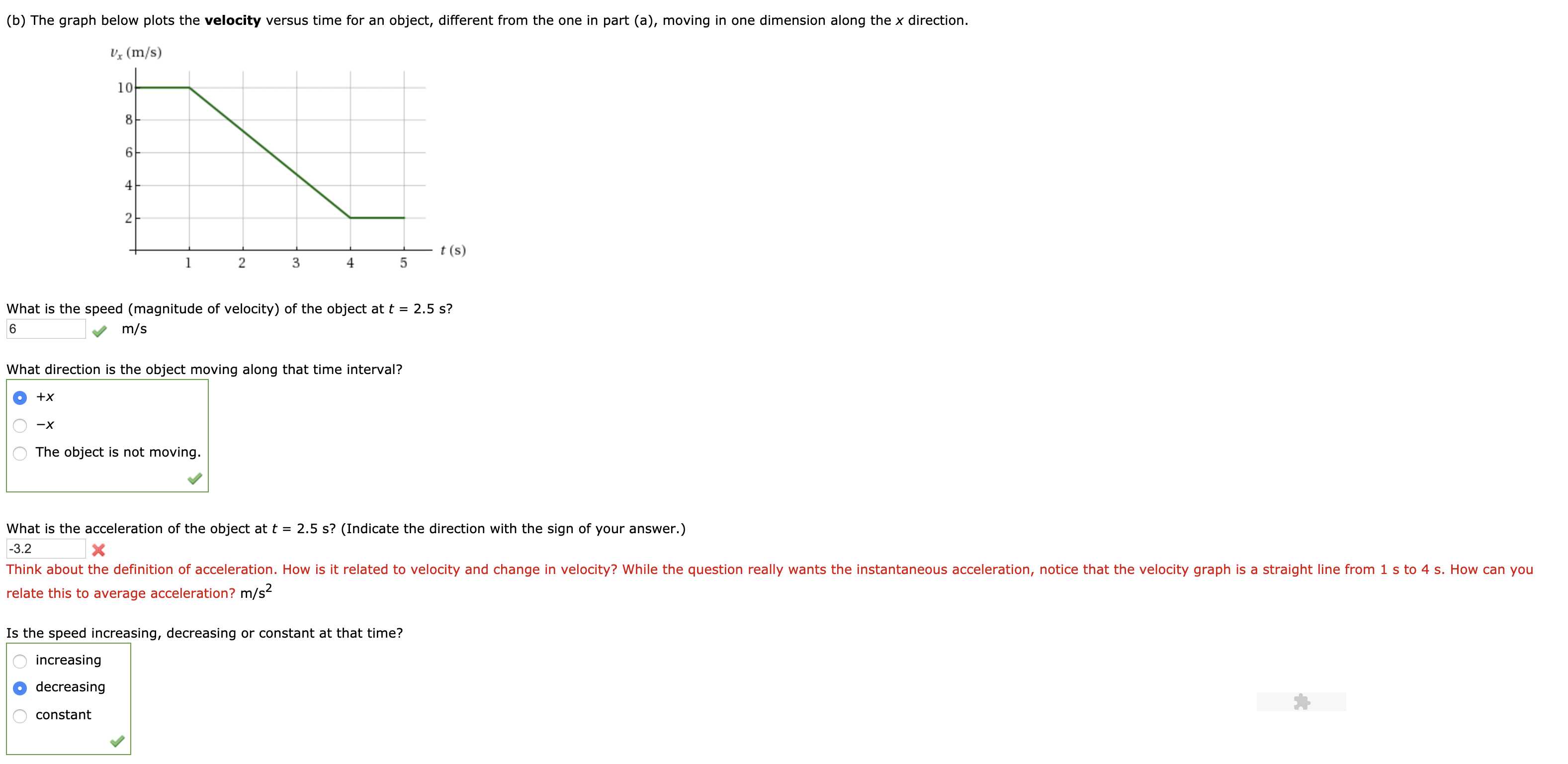# (b) The graph below plots the velocity versus time for an object, different from the one in part (a), moving in one dimension along the x direction. Vx (m/s) 10 2 t (s) 3 4 What is the speed (magnitude of velocity) of the object at t-2.5 s? 6 m/s What direction is the object moving along that time interval? Онх The object is not moving what is the acceleration of the object at t = 2.5 s? (Indicate the direction with the sign of your answer.) 3.2 Think about the definition of acceleration. How is it related to velocity and change in velocity? While the question really wants the instantaneous acceleration, notice that the velocity graph is a straight line from 1 s to 4 s. How can you relate this to average acceleration? m/s Is the speed increasing, decreasing or constant at that time? increasing decreasing constant

Questionhelp_outlineImage Transcriptionclose(b) The graph below plots the velocity versus time for an object, different from the one in part (a), moving in one dimension along the x direction. Vx (m/s) 10 2 t (s) 3 4 What is the speed (magnitude of velocity) of the object at t-2.5 s? 6 m/s What direction is the object moving along that time interval? Онх The object is not moving what is the acceleration of the object at t = 2.5 s? (Indicate the direction with the sign of your answer.) 3.2 Think about the definition of acceleration. How is it related to velocity and change in velocity? While the question really wants the instantaneous acceleration, notice that the velocity graph is a straight line from 1 s to 4 s. How can you relate this to average acceleration? m/s Is the speed increasing, decreasing or constant at that time? increasing decreasing constant fullscreen

### Want to see this answer and more?

Experts are waiting 24/7 to provide step-by-step solutions in as fast as 30 minutes!*